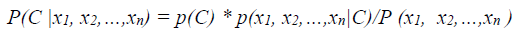## Classification

Text classification is the task of classifying documents based on their contents (Kamruzzaman et al. 2005). Researchers have considered several approaches of text classification.
Kamruzaman, Farhana Haider and Ahmed Ryadh Hassan (2005) used Naïve Bayes to classify document. Bijalwan et al. (2014) used k-NN and Naïve Bayes to categorize the documents. They concluded that k-NN works better than Naïve Bayes on their data set. Beriman (2001) mentioned Random Forest is effective tool for prediction.

## Naïve Bayes Classifier

The Naïve Bayes classifier is a simple classifier that can be very accurate. Naïve Bayes classifier has a statistical method where the frequency of the words makes this prediction. In this classification, each category of words will have its probability, which calculate based on the terms in the training documents (Bijalwan et al. 2014). Bijalwan et al. (2014) also mentions “They are called “naive” because the algorithm assumes that all terms occur independent from each other.”
Let c be the class andare the predictors of c then Naïve Bayes classifier equation is as follows:1

P(C|) is the posterior probability of class C. P(C) is the probability of class C. P(|C) is the probability of predictor in class C and P() is the probability of each predictor (Saedsayed 2015).

## Random Forest

Random forest is the combination of decision tress where all the decision tress have the same distribution. Kamruzzamn and others (2005) and Kotsiantis (2007) demonstrated the accuracy of decision tree for classification. Decision tree builds classification in the form of tree. This algorithm breaks down data set to small subsets that are fit with decision trees. The multiple decision trees vote to determine the class of new records (Sci-kit Learn 2015b).

## k-Nearest Neighbor (kNN) Classifier

The basic idea comes from the K nearest document that are around the category of a given query in the document space (Bijalwan et al. 2014). Figure 2 shows the k-NN classifier.Figure 2. K-NN classifier (Wikipedia 2015)

The k-nearest neighbors returns the average values of the k-th nearest tuples. The equation on K-NN is as follow:This classification would ID the nearest n record and classify the record based on the most common features.